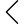648 products found
• s
• m
• l
• xl
• s
• m
• l
• xl
• s
• m
• l
• xl
• s
• m
• l
• xl
• s
• m
• l
• xl
• s
• m
• l
• xl
Utility GPC Work Pants
\$429.00 \$343.20
• s
• m
• l
• xl
• s
• m
• l
• xl
• s
• m
• l
• xl
• s
• m
• l
• xl
• s
• m
• l
• xl
• 2xl
\$189.00 \$132.30
• s
• m
• l
• xl
Geo knitted lounge shorts
\$189.00 \$141.75
• s
• m
• l
• xl
GEO-CUT SAROUEL SHORTS
\$199.00 \$59.70
• s
• m
• l
• xl
mesh GP drawstring pullover
\$199.00 \$169.15
• s
• m
• l
• xl
• s
• m
• l
• xl
• s
• m
• l
• xl
• s
• m
• l
• xl
• 2xl
• s
• m
• l
• xl
• s
• m
• l
• xl
• s
• m
• l
• xl
• s
• m
• l
• xl
• s
• m
• l
• xl
• s
• m
• l
• xl
• s
• m
• l
• xl
ANTARES / MTD Cargo pants
\$459.00 \$367.20
• s
• m
• l
• xl
• s
• m
• l
• xl
• s
• m
• l
• xl
• s
• m
• l
• xl
• s
• m
• l
• xl
Geo knitted lounge shorts
\$189.00 \$141.75
• s
• m
• l
• xl
• s
• m
• l
• xl
• s
• m
• l
• xl
• s
• m
• l
• xl
• s
• m
• l
• xl
• s
• m
• l
• xl
• s
• m
• l
• xl
lpu / geo-panel overcoat
\$399.00 \$299.25
• s
• m
• l
• xl
• s-m
• l-xl
•• 1
• 2
• 3
• 17